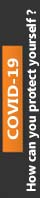2019-02-01T10:38:47+00:00 essay assignments

# Genetic Variation, Genotypes, and Disease

This paper concentrates on the primary theme of Genetic Variation, Genotypes, and Disease in which you have to explain and evaluate its intricate aspects in detail. In addition to this, this paper has been reviewed and purchased by most of the students hence; it has been rated 4.8 points on the scale of 5 points. Besides, the price of this paper starts from £ 79. For more details and full access to the paper, please refer to the site.

# Genetic Variation, Genotypes, and Disease

1 Define the term "genetic variation."

If a gene or locus has two alleles (A and a) in a population, what are all the possible genotypes?

If the frequency of (A) allele = p, and the frequency of (a) allele is q, what is the frequency of all possible genotypes in this population?

2 What is the Hardy-Weinberg equilibrium?

In a population, a locus A has two alleles (A) and (a). The frequency f of (A) is f (A) = 0.6; what is the f (a)?

Using these frequencies, calculate the frequencies of all possible genotypes in a population in Hardy-Weinberg equilibrium.

3 In a population (Z), the frequencies of genotypes of a two allele locus (B and b) are f(BB) = 0.3, f(bb) = 0.6, f (Bb) = 0.1. Calculate the frequencies of both alleles.

Using the allele frequencies calculated in a., calculate the frequencies of all possible genotypes at this locus in a population after one generation of random mating.

Is the population (Z) in part a above in Hardy-Weinberg equilibrium? The population size is 1000.

4 Describe how you would use the Hardy-Weinberg equilibrium to calculate genotype frequencies of a locus with three alleles.
If the f(D) of an X-linked gene in a population = 0.8, what is the f(d) of the other allele at this locus?

What is the frequency of females` homozygous for d allele (XdXd)?

What is the frequency of males` hemizygous for the d allele (XdY)?

5 Explain why more males present with X-linked recessive diseases. Show your reasoning.

Why must the five assumptions/criteria apply to a population before we can say it is in Hardy-Weinberg equilibrium?

Take any one of these assumptions and explain in detail how it could disrupt the Hardy-Weinberg equilibrium for a particular gene locus.

0% Plagiarism Guaranteed & Custom Written, Tailored to your instructions

International House, 12 Constance Street, London, United Kingdom,
E16 2DQ

## STILL NOT CONVINCED?

We've produced some samples of what you can expect from our Academic Writing Service - these are created by our writers to show you the kind of high-quality work you'll receive. Take a look for yourself!FLAT 25% OFF ON EVERY ORDER.Use "FLAT25" as your promo code during checkout